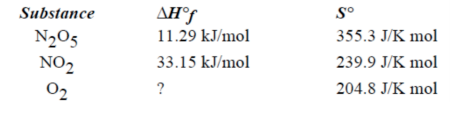# Problem: Consider the reaction of N2O5 at 25°C for which the following data are relevant:2 N2O5 (g)      ⇌       4 NO 2 (g) + O2 (g)What is the ΔH° for the reaction?A. 110.02 kJB. 21.86 kJC. -21.86 kJD. 155.20 kJE. -155.20 kJ

###### FREE Expert Solution
95% (310 ratings)
###### Problem Details

Consider the reaction of N2O5 at 25°C for which the following data are relevant:

2 N2O5 (g)      ⇌       4 NO 2 (g) + O2 (g)

What is the ΔH° for the reaction?

A. 110.02 kJ

B. 21.86 kJ

C. -21.86 kJ

D. 155.20 kJ

E. -155.20 kJWhat scientific concept do you need to know in order to solve this problem?

Our tutors have indicated that to solve this problem you will need to apply the Enthalpy of Formation concept. You can view video lessons to learn Enthalpy of Formation. Or if you need more Enthalpy of Formation practice, you can also practice Enthalpy of Formation practice problems.

What is the difficulty of this problem?

Our tutors rated the difficulty ofConsider the reaction of N2O5 at 25°C for which the followin...as low difficulty.

How long does this problem take to solve?

Our expert Chemistry tutor, Jules took 2 minutes and 18 seconds to solve this problem. You can follow their steps in the video explanation above.

What professor is this problem relevant for?

Based on our data, we think this problem is relevant for Professor Halihan's class at OKSTATE.